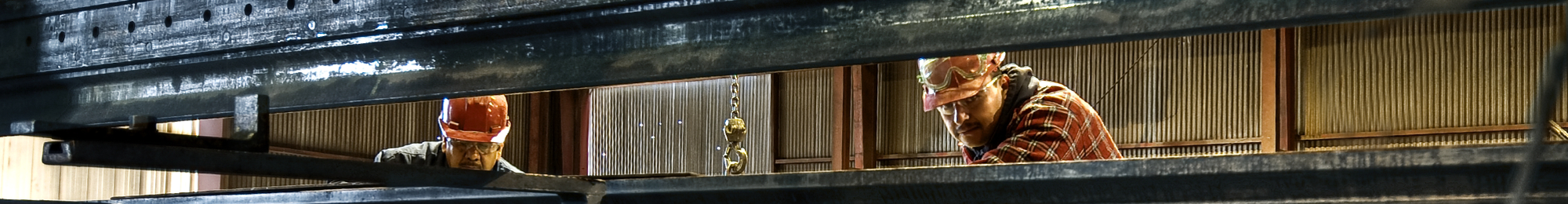Willbanks Metals# Steel Product Sizing & Calculations

### CIRCLE

Area = Square of Diameter x .7854 or Square of Radius x 3.1416

Circumference = Diameter x 3.1416

Diameter = Circumference x .3183Doubling diameter increases area four times; tripling diameter increases area nine times, etc.

### SQUARE

Area = Square of Side

Diagonal = Side x 1.4142

Side = Diagonal x .7071

### SQUARE INSCRIBED IN CIRCLE

Side of Square = Diameter of Circle x .7071 or Circumference of Circle x .2251

Diameter of Circle = Side of Square x 1.4142

Circumference of Circle = Side of Square x 4.4429

### SQUARE AND CIRCLE WITH EQUAL AREA

Side of Square = Diameter of Circle x .8862

Diameter of Circle = Side of Square 1.128

Circumference of Circle = side of Square x 3.545

### RECTANGLE

Area = Length x Width

Diagonal = Square root of sum of squares of Width and Length

### TRIANGLE

Area = Base x 1/2 of Perpendicular Height

### HEXAGON (Equal sides and angles)

Area = Square of Distance across Flats x .866 or Square of Side x 2.598

Side = 1/2 of Diagonal or Distance across Flats x .577

Diagonal = Distance across Flats x 1.155 or Side x 2

### OCTAGON (Equal sides and angles)

Area = Square of Distance across Flats x .828 or Square of Side x 4.828

Side = Diagonal x .383 or Distance across Flats x 4.14

Diagonal = Distance across Flats x 1.082 or Side x 2.613

### SPHERE

Area of Surface = Square of Diameter x 3.1416

Volume = Cube of Diameter x .5236

### CUBE

Area of Surface = Square of Side x 6

Volume = Cube of Side

Diagonal = Side x 1.732

### CYLINDER

Area of curved Surface – Diameter x Length 3.1416

Volume = Square of Diameter x Length x .785

### CONE

Area of Curved Surface = Diameter of Base x Slant Height x 1.5708

Volume = Diameter of Base Squared x Perpendicular Height x .2618 or Area of Base x 1/3 Perpendicular Height

### PYRAMID

Lateral Surface Area = (not incl. base) = Perimeter of Base x 1/2 Slant Height

Volume = Area of Base x 1/3 Perpendicular Height